5/19/2015

## Intro

Recall from Chapter 1 that categorical variables divide our data into groups (or categories).

Consider a data set containing descriptions of the 1313 passengers aboard The Titanic. The data looks like this:

##                                  Name PClass   Age    Sex Survived
## 1        Allen, Miss Elisabeth Walton    1st adult female    alive
## 2         Allison, Miss Helen Loraine    1st child female     dead
## 5       Allison, Master Hudson Trevor    1st child   male    alive
## 6                  Anderson, Mr Harry    1st adult   male    alive

Which variables are categorical?

## 2.1 Summarizing a Single Categorical Variable

The problem with looking at the raw data is that we can't see what's going on.

The goal of summarizing the data is to see how the levels of the variables are distributed across our observations.

The distribution of the variables can help us answer questions about our data.

• Were first class passengers more or less likely to die than the other classes?
• Was "women and children first" really followed on The Titanic?
• Is there a relationship between class and gender when it came to surviving (e.g., were first class women more likely to survive than third class women)?

## Frequency Tables

The simplest way to summarize a single categorical variable is by counting the levels. We call tables of counts frequency tables.

Frequency tables for class:

Class Frequency
1st $$322$$
2nd $$280$$
3rd $$711$$
Total $$1313$$

## Relative Frequencies

Another option is to look at the sample proportion or relative frequency of observations with a particular label. A table of these proportions is called a relative frequency table.

Let $$x$$ represent our count, $$n$$ represent our number of individuals, and $$\hat{p}$$ represent the sample proportion. Any given sample proportion can be calculated as:

$\hat{p} = \frac{x}{n}$

So, for example, the proportion of first class passenger is found as:

$\hat{p} = \frac{322}{1313} = 0.245$

## Relative Frequency Tables

If we calculate the proportions of all of the levels of a variable, we can create a relative frequency table. For the passenger classes:

Class Relative Frequency
1st $$0.245$$
2nd $$0.213$$
3rd $$0.542$$
Total $$1$$

Note that within a variable, all relative frequencies must add up to exactly one because every individual needs to have a label.

## Percentages

We can also represent these proportions as percentages by multiplying each one by 100.

Class Percentage
1st $$24.5$$
2nd $$21.3$$
3rd $$54.2$$
Total $$100$$

In this case, all percentages must add up to 100.

## Contingency Tables

To find relationships between variables, we compare the distribution of one variable within each of the levels of another variable. We call this conditioning a variable.

For example, we can look at the relationship between Survived and PClass:

Class/Survived 1st 2nd 3rd
Alive 193 119 138

Does this say anything about the relationship between the passengers' class and their chances for survival?

## Visualizing Categorical Data

There are many types of graphs for displaying categorical variables, but we will focus on two of them:

• Bar Charts
• Pie Charts

In either case, the graph should be a quick way of visualizing our variables and seeing the distribution.

No matter what graph we choose, the visual representation should follow the area principle:

• The area a visual representation takes up on the graph should correspond to the magnitude (size) of the value it represents.

## Bar Charts

In a bar chart:

• The categories are on one axis and the frequencies (or relative frequencies) on the other
• Bars are drawn perpendicular to the category axis
• The heights of the bars correspond to the frequencies

Bar charts can have a vertical or horizontal layout, but the basic structure remains the same.

Whether we plot frequencies or relative frequencies, the graph looks basically the same. The only difference is the scale of the axis.

We can also stack multiple categories on top of or next to each other to find relationships between variables.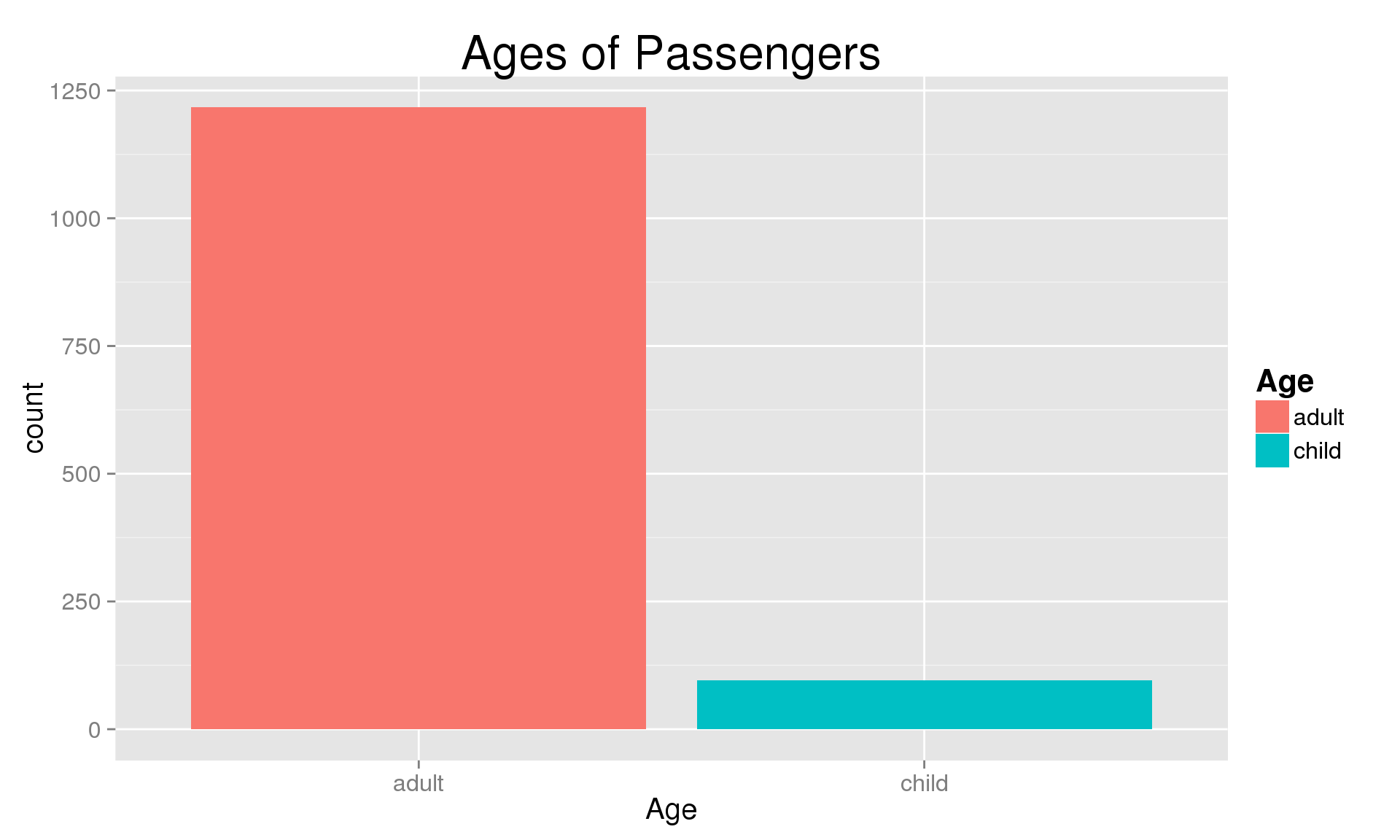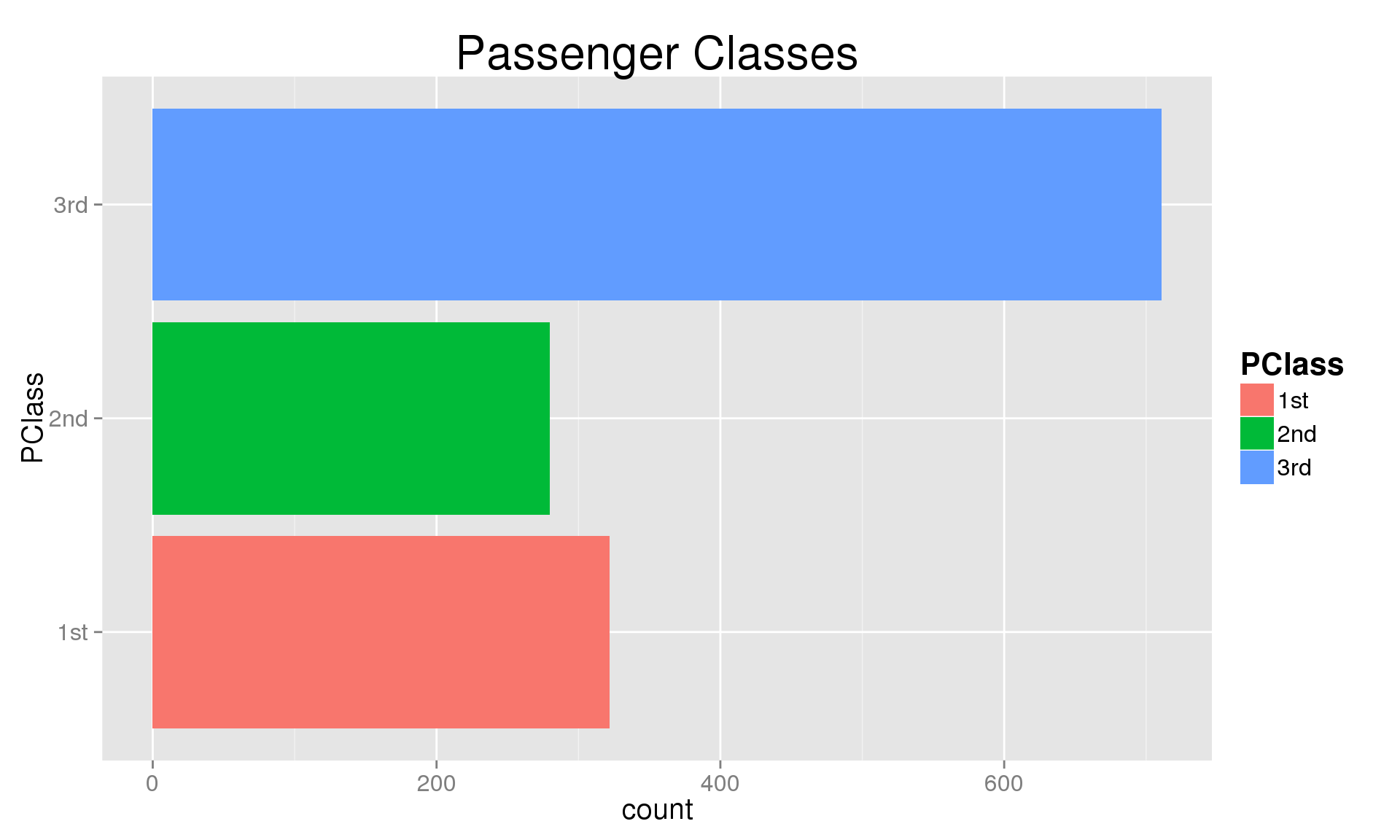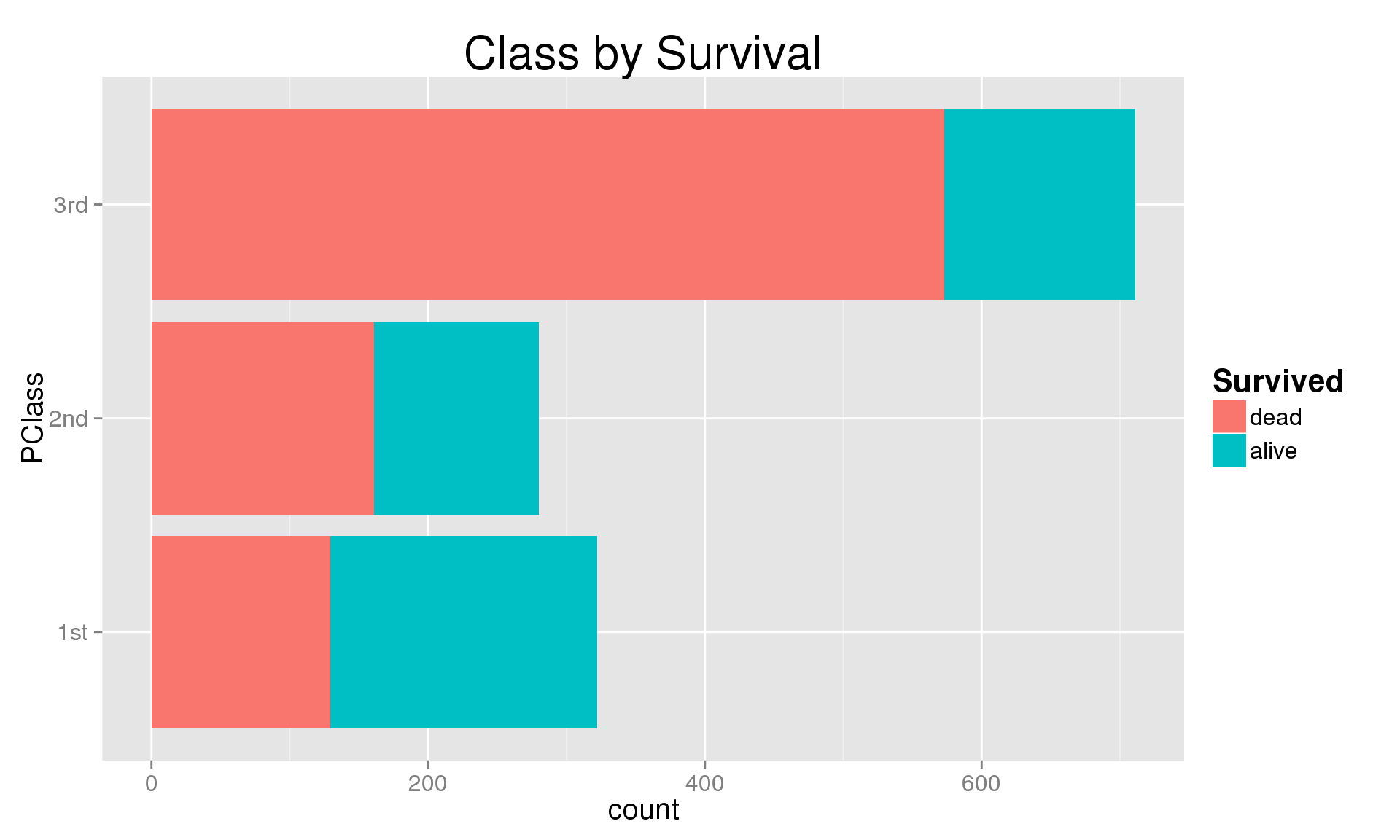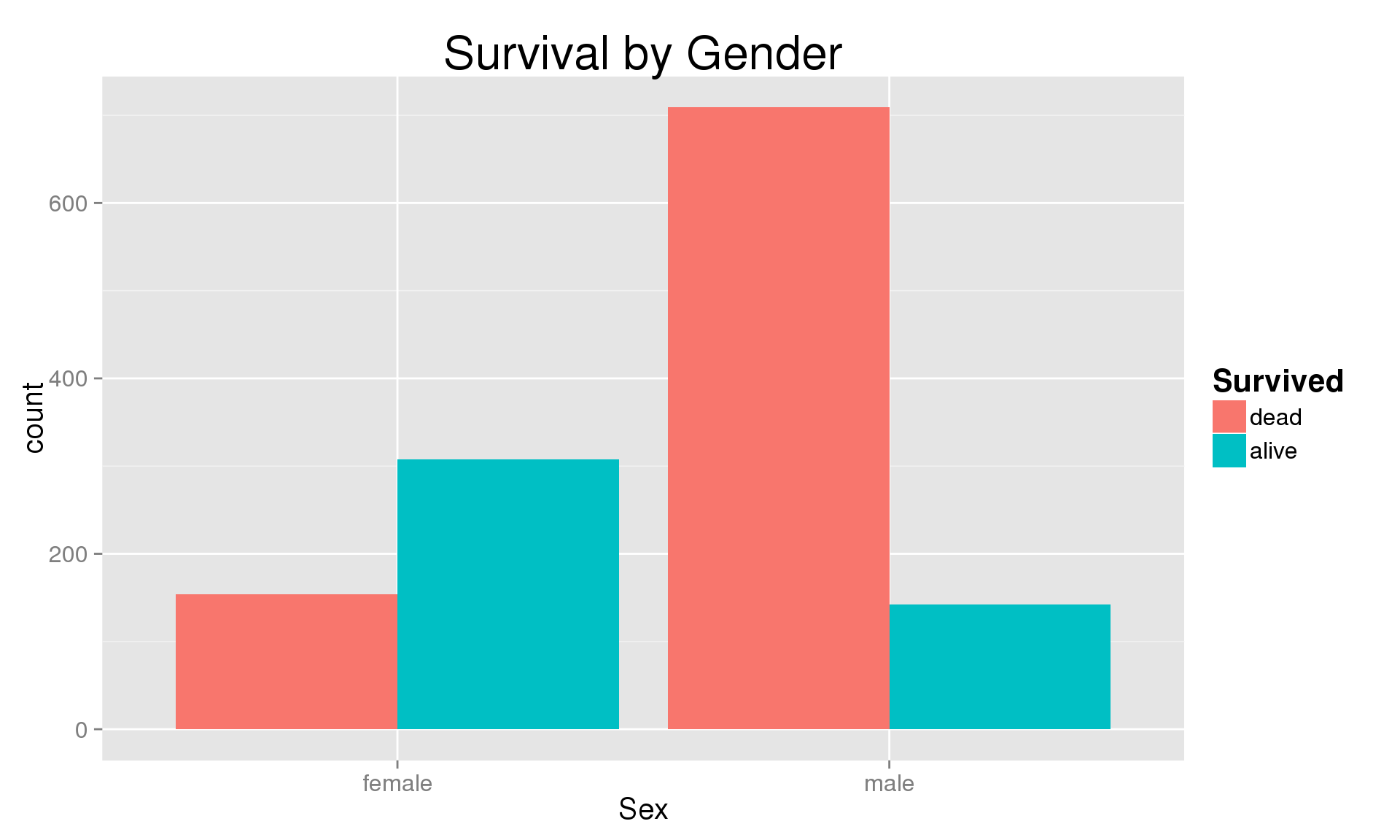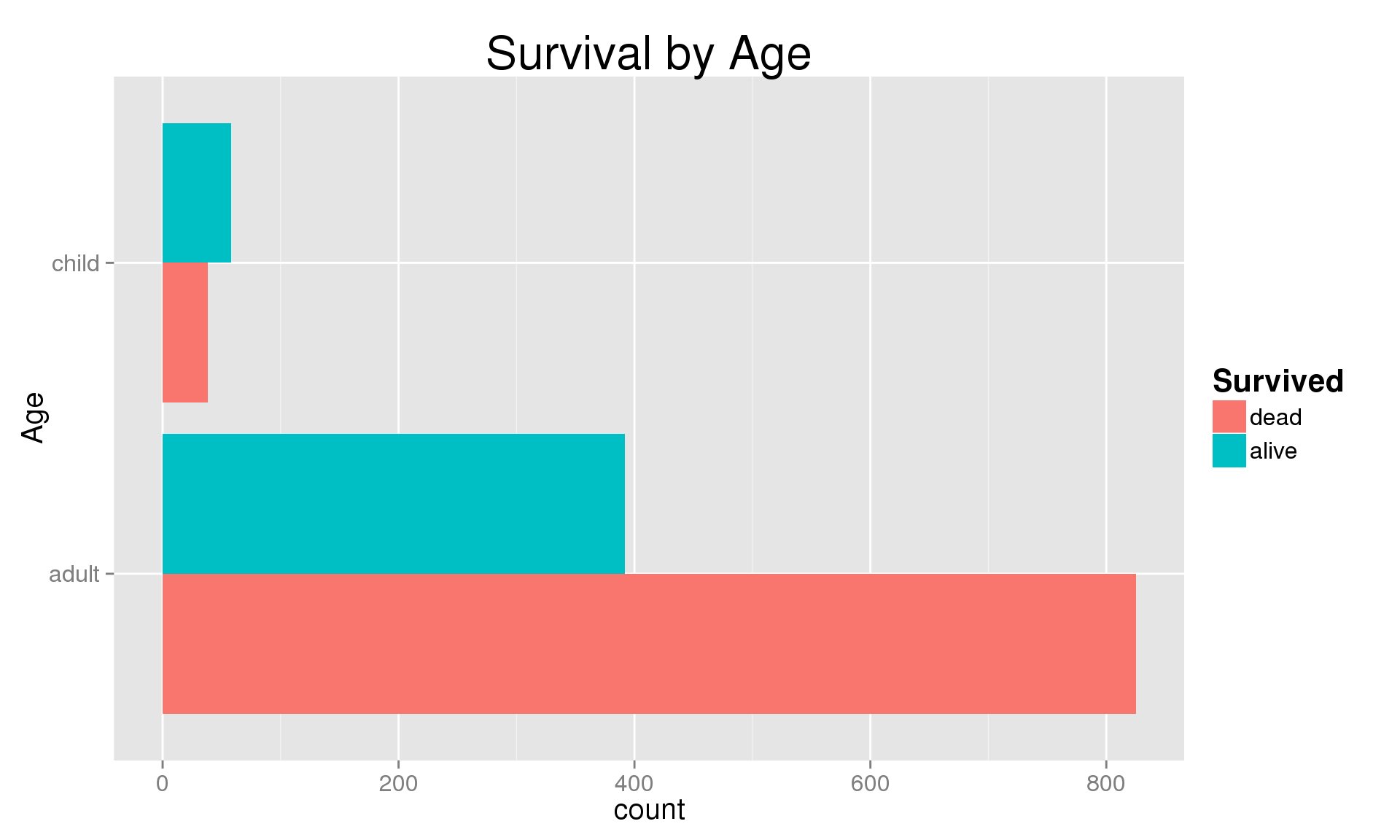## Pie Charts

Pie charts visualize categorical variables by graphing them as sections of a circle

• The area of the "pie slice" is proportional to the relative frequency (either proportions or percentages)
• The areas of the pie slices must add up to 1 (or 100%)
• Each observation must fit into exactly one category

Downsides:

• Humans are extraordinarily bad perceiving smaller differences in the areas
• It's hard to translate from areas of a slice to percentages, proportions, or counts without explicit labels.
• Comparing groups means multiple charts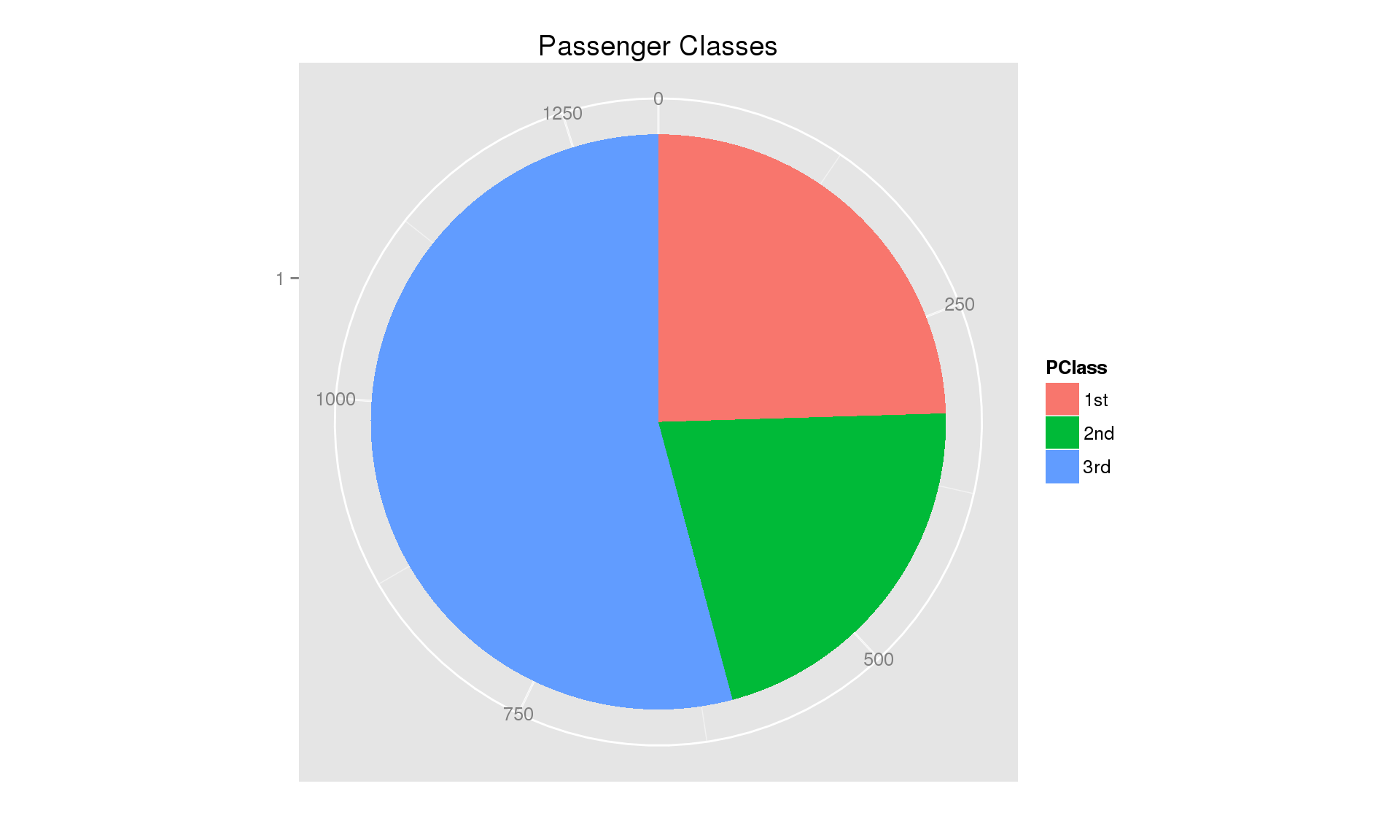## Comparing Sex and Survival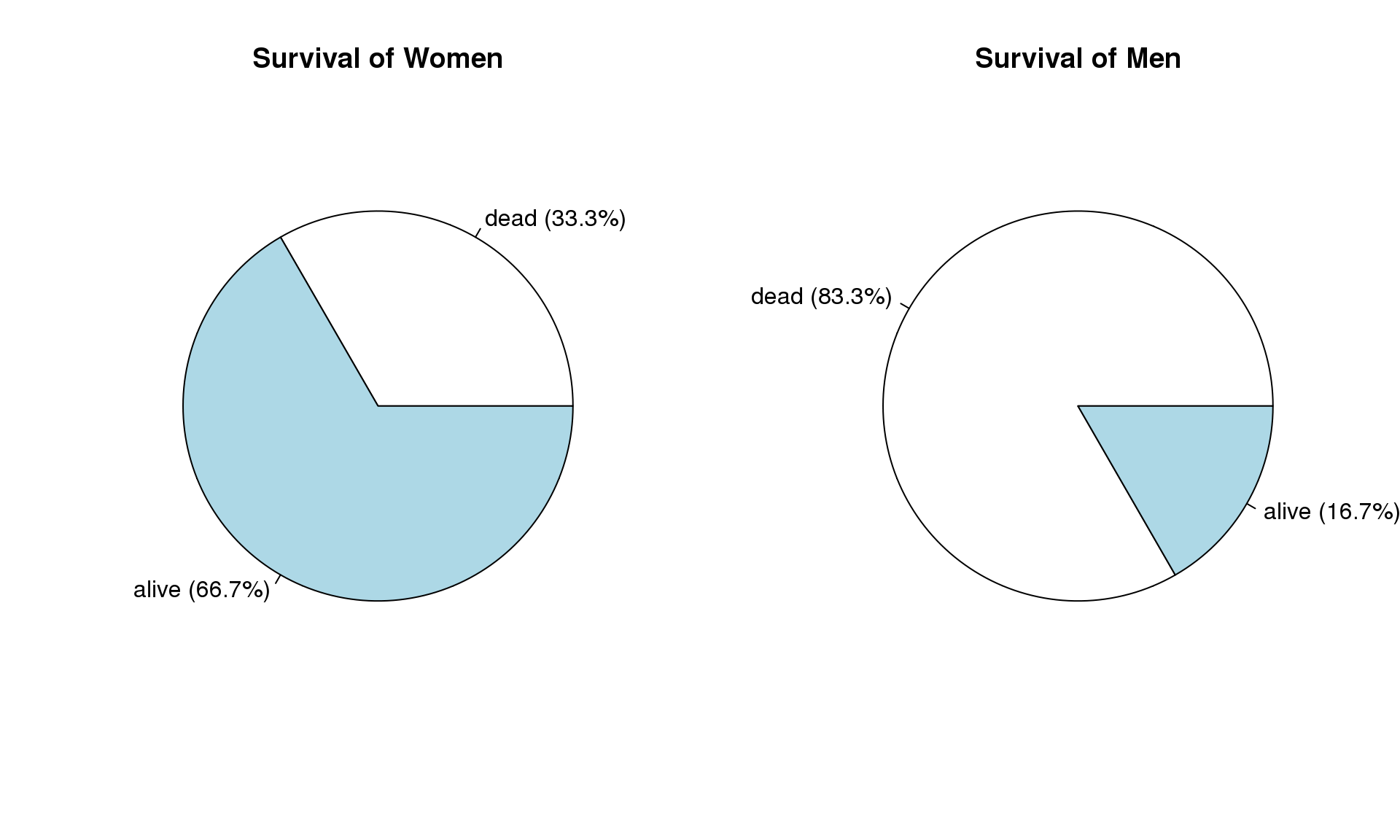Notice:

• We can't really compare the genders of the survivors or the dead
• I.e., there were many more men, so there may still be more male survivors than female.
• The only way to know for sure would be to look at the counts, or invert the chart and graph the percentages of gender within alive and dead.

## Comparing Sex and Survival (V2)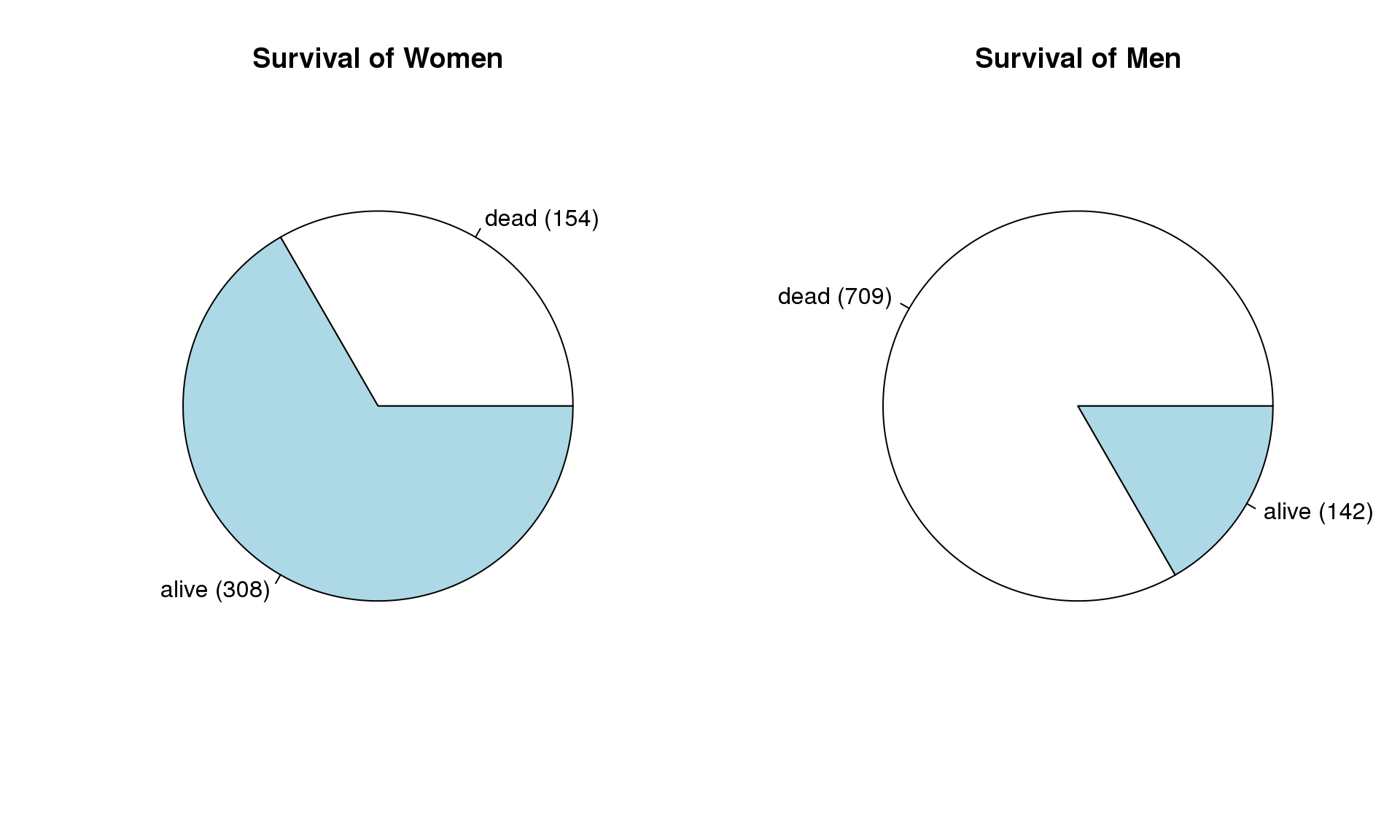## Practice

In a group of thirty-one students twenty have brown eyes, eight have blue eyes, and three have hazel eyes find:

• The frequency of students with hazel eyes $$(x_h)$$
• The relative frequency of students with brown eyes $$(\hat{p}_{br})$$
• The percentage of students with blue eyes $$(100 \times \hat{p}_{bl})$$

Solutions:

• $$x_{h} = 3$$
• $$\hat{p}_{br} = \frac{x_{br}}{n} = \frac{20}{31} = 0.645$$
• $$100\times \hat{p}_{bl} = 100\times\frac{x_{bl}}{n} = 100\times\frac{3}{31} = 100\times 0.258 = 25.8\%$$

## Practice (Cont.)

Using the same data as before, the summary tables are:

Eye Color Frequency Rel. Freq Percentage
Brown $$20$$ $$0.645$$ $$64.5\%$$
Blue $$8$$ $$0.258$$ $$25.8\%$$
Hazel $$3$$ $$0.097$$ $$9.7\%$$
Total $$n = 31$$ $$1$$ $$100\%$$

Graph in Statcrunch

## Summary

Numerical summaries for categorical variables include:

• Frequencies/Counts
• Relative Frequencies/Proportions
• Percentages

Visual Summaries (graphs) include:

• Bar Graphs
• Pie Charts